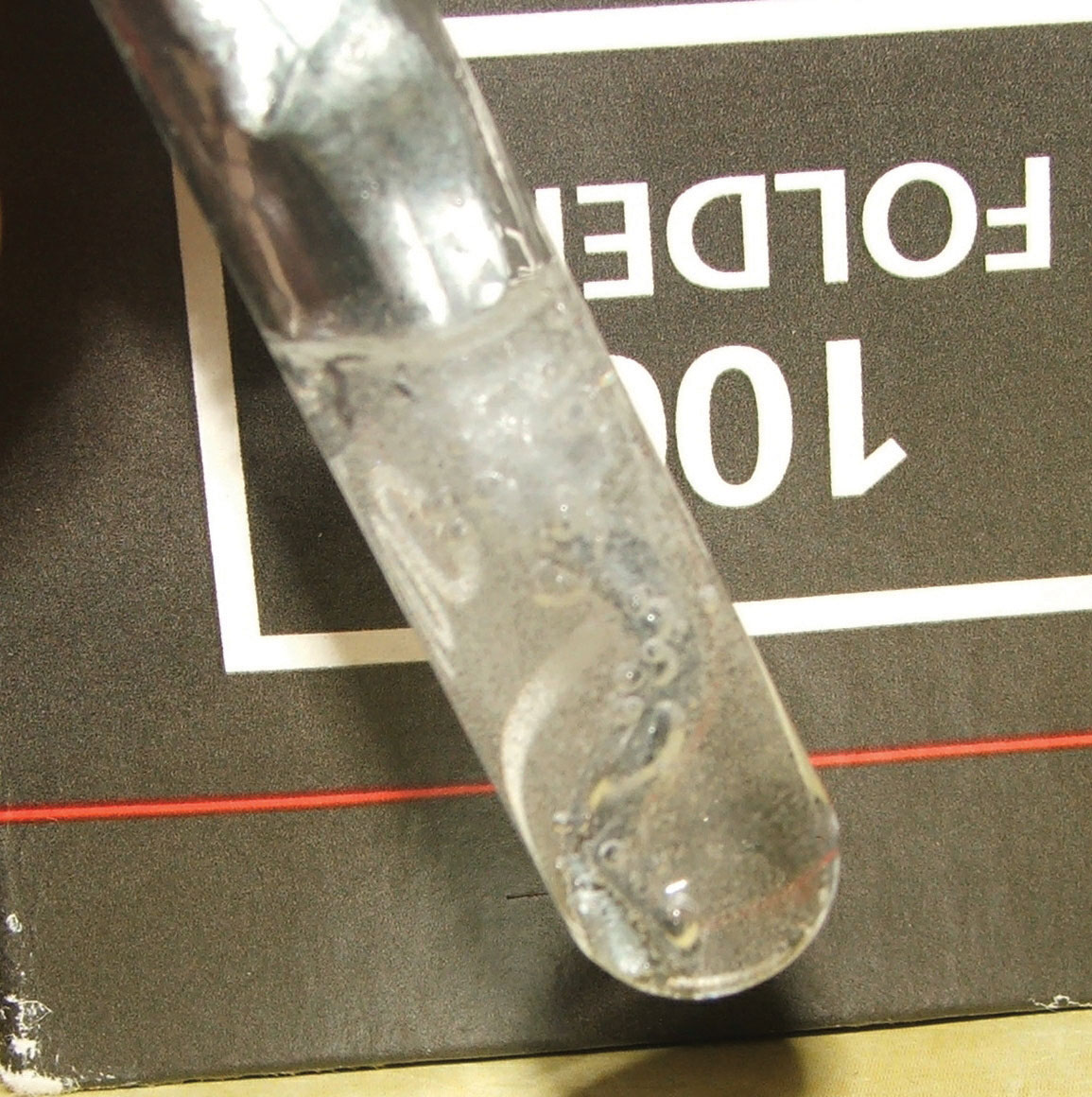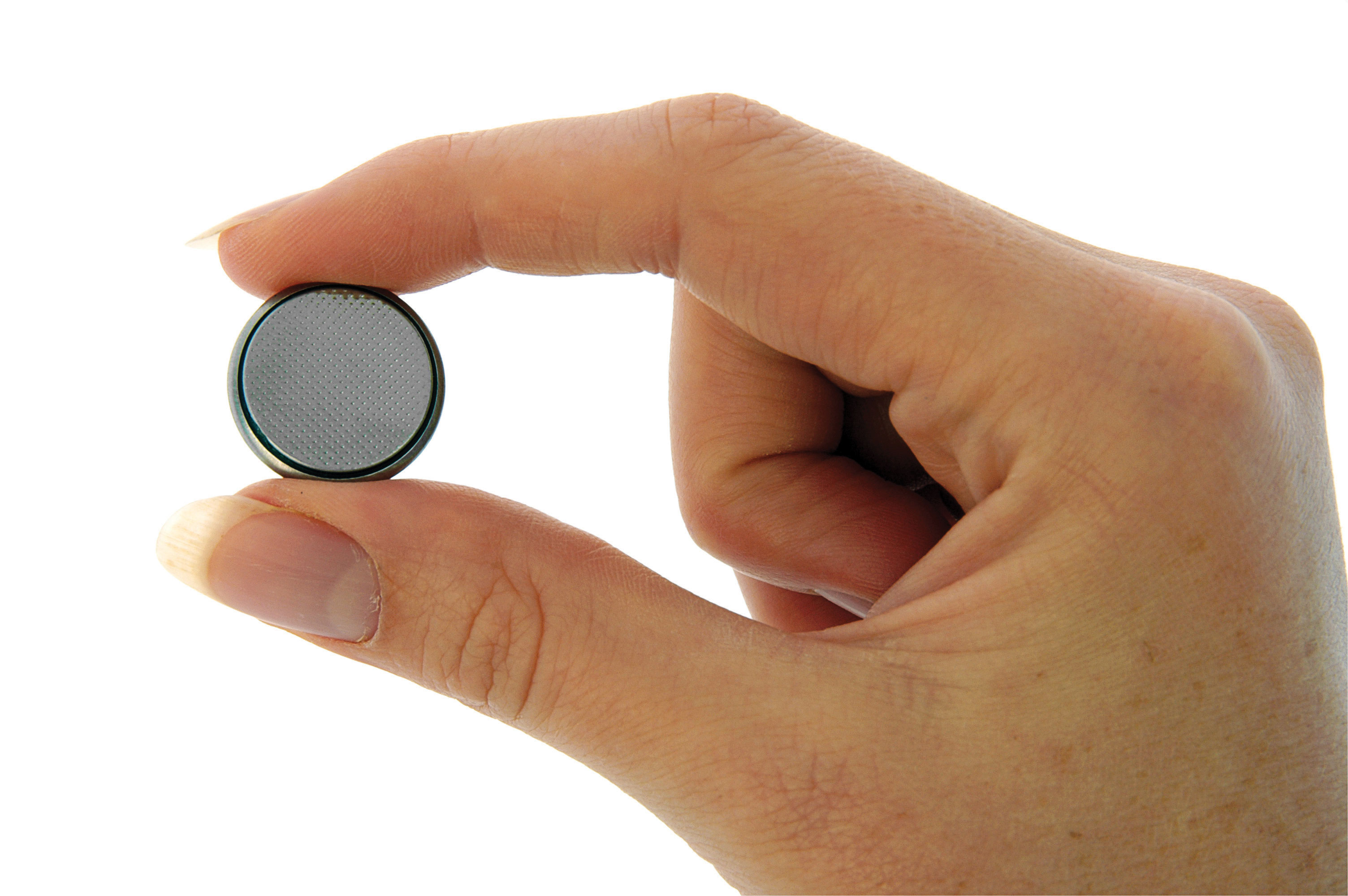## 5.5 Oxidation-Reduction (Redox) Reactions

### Learning Objective

1. Identify a chemical reaction as an oxidation-reduction reaction.

Figure 5.4 Zinc Metal plus Hydrochloric AcidIt is fairly obvious that zinc metal reacts with aqueous hydrochloric acid! The bubbles are hydrogen gas.

When zinc metal is submerged into a quantity of aqueous HCl, the following reaction occurs (Figure 5.4 "Zinc Metal plus Hydrochloric Acid"):

Zn(s) + 2HCl(aq) → H2(g) + ZnCl2(aq)

This is one example of what is sometimes called a single replacement reaction because Zn replaces H in combination with Cl.

Because some of the substances in this reaction are aqueous, we can separate them into ions:

Zn(s) + 2H+(aq) + 2Cl(aq) → H2(g) + Zn2+(aq) + 2Cl(aq)

Viewed this way, the net reaction seems to be a charge transfer between zinc and hydrogen atoms. (There is no net change experienced by the chloride ion.) In fact, electrons are being transferred from the zinc atoms to the hydrogen atoms (which ultimately make a molecule of diatomic hydrogen), changing the charges on both elements.

To understand electron-transfer reactions like the one between zinc metal and hydrogen ions, chemists separate them into two parts: one part focuses on the loss of electrons, and one part focuses on the gain of electrons. The loss of electrons is called oxidationThe loss of electrons by an atom.. The gain of electrons is called reductionThe gain of electrons by an atom.. Because any loss of electrons by one substance must be accompanied by a gain in electrons by something else, oxidation and reduction always occur together. As such, electron-transfer reactions are also called oxidation-reduction reactionsA chemical reaction in which electrons are transferred from one atom to another. Also called a redox reaction., or simply redox reactions. The atom that loses electrons is oxidized, and the atom that gains electrons is reduced. Also, because we can think of the species being oxidized as causing the reduction, the species being oxidized is called the reducing agentA species that causes reduction, which is itself oxidized., and the species being reduced is called the oxidizing agentA species that causes oxidation, which is itself reduced..

### Note

Because batteries are used as sources of electricity (that is, of electrons), all batteries are based on redox reactions.

Although the two reactions occur together, it can be helpful to write the oxidation and reduction reactions separately as half reactionsA chemical reaction that shows only oxidation or reduction.. In half reactions, we include only the reactant being oxidized or reduced, the corresponding product species, any other species needed to balance the half reaction, and the electrons being transferred. Electrons that are lost are written as products; electrons that are gained are written as reactants. For example, in our earlier equation, now written without the chloride ions,

Zn(s) + 2H+(aq) → Zn2+(aq) + H2(g)

zinc atoms are oxidized to Zn2+. The half reaction for the oxidation reaction, omitting phase labels, is as follows:

Zn → Zn2+ + 2e

This half reaction is balanced in terms of the number of zinc atoms, and it also shows the two electrons that are needed as products to account for the zinc atom losing two negative charges to become a 2+ ion. With half reactions, there is one more item to balance: the overall charge on each side of the reaction. If you check each side of this reaction, you will note that both sides have a zero net charge.

Hydrogen is reduced in the reaction. The balanced reduction half reaction is as follows:

2H+ + 2e → H2

There are two hydrogen atoms on each side, and the two electrons written as reactants serve to neutralize the 2+ charge on the reactant hydrogen ions. Again, the overall charge on both sides is zero.

The overall reaction is simply the combination of the two half reactions and is shown by adding them together.Because we have two electrons on each side of the equation, they can be canceled. This is the key criterion for a balanced redox reaction: the electrons have to cancel exactly. If we check the charge on both sides of the equation, we see they are the same—2+. (In reality, this positive charge is balanced by the negative charges of the chloride ions, which are not included in this reaction because chlorine does not participate in the charge transfer.)

Redox reactions are often balanced by balancing each individual half reaction and then combining the two balanced half reactions. Sometimes a half reaction must have all of its coefficients multiplied by some integer for all the electrons to cancel. The following example demonstrates this process.

### Example 5

Write and balance the redox reaction that has silver ions and aluminum metal as reactants and silver metal and aluminum ions as products.

Solution

We start by using symbols of the elements and ions to represent the reaction:

Ag+ + Al → Ag + Al3+

The equation looks balanced as it is written. However, when we compare the overall charges on each side of the equation, we find a charge of +1 on the left but a charge of +3 on the right. This equation is not properly balanced. To balance it, let us write the two half reactions. Silver ions are reduced, and it takes one electron to change Ag+ to Ag:

Ag+ + e → Ag

Aluminum is oxidized, losing three electrons to change from Al to Al3+:

Al → Al3+ + 3e

To combine these two half reactions and cancel out all the electrons, we need to multiply the silver reduction reaction by 3:Now the equation is balanced, not only in terms of elements but also in terms of charge.

### Skill-Building Exercise

1. Write and balance the redox reaction that has calcium ions and potassium metal as reactants and calcium metal and potassium ions as products.

### Note

Potassium has been used as a reducing agent to obtain various metals in their elemental form.

### To Your Health: Redox Reactions and Pacemaker Batteries

All batteries use redox reactions to supply electricity because electricity is basically a stream of electrons being transferred from one substance to another. Pacemakers—surgically implanted devices for regulating a person’s heartbeat—are powered by tiny batteries, so the proper operation of a pacemaker depends on a redox reaction.

Pacemakers used to be powered by NiCad batteries, in which nickel and cadmium (hence the name of the battery) react with water according to this redox reaction:

Cd(s) + 2NiOOH(s) + 2H2O(ℓ) → Cd(OH)2(s) + 2Ni(OH)2(s)

The cadmium is oxidized, while the nickel atoms in NiOOH are reduced. Except for the water, all the substances in this reaction are solids, allowing NiCad batteries to be recharged hundreds of times before they stop operating. Unfortunately, NiCad batteries are fairly heavy batteries to be carrying around in a pacemaker. Today, the lighter lithium/iodine battery is used instead. The iodine is dissolved in a solid polymer support, and the overall redox reaction is as follows:

2Li(s) + I2(s) → 2LiI(s)

Lithium is oxidized, and iodine is reduced. Although the lithium/iodine battery cannot be recharged, one of its advantages is that it lasts up to 10 years. Thus, a person with a pacemaker does not have to worry about periodic recharging; about once per decade a person requires minor surgery to replace the pacemaker/battery unit. Lithium/iodine batteries are also used to power calculators and watches.A small button battery like this is used to power a watch, pacemaker, or calculator.

Oxidation and reduction can also be defined in terms of changes in composition. The original meaning of oxidation was “adding oxygen,” so when oxygen is added to a molecule, the molecule is being oxidized. The reverse is true for reduction: if a molecule loses oxygen atoms, the molecule is being reduced. For example, the acetaldehyde (CH3CHO) molecule takes on an oxygen atom to become acetic acid (CH3COOH).

2CH3CHO + O2 → 2CH3COOH

Thus, acetaldehyde is being oxidized.

Similarly, oxidation and reduction can be defined in terms of the gain or loss of hydrogen atoms. If a molecule adds hydrogen atoms, it is being reduced. If a molecule loses hydrogen atoms, the molecule is being oxidized. For example, in the conversion of acetaldehyde into ethanol (CH3CH2OH), hydrogen atoms are added to acetaldehyde, so the acetaldehyde is being reduced:

CH3CHO + H2 → CH3CH2OH

### Example 6

In each conversion, indicate whether oxidation or reduction is occurring.

1. N2 → NH3
2. CH3CH2OHCH3 → CH3COCH3
3. HCHO → HCOOH

Solution

1. Hydrogen is being added to the original reactant molecule, so reduction is occurring.
2. Hydrogen is being removed from the original reactant molecule, so oxidation is occurring.
3. Oxygen is being added to the original reactant molecule, so oxidation is occurring.

### Skill-Building Exercise

In each conversion, indicate whether oxidation or reduction is occurring.

1. CH4 → CO2 + H2O

2. NO2 → N2

3. CH2=CH2 → CH3CH3

### Concept Review Exercises

1. Give two different definitions for oxidation and reduction.

2. Give an example of each definition of oxidation and reduction.

1. Oxidation is the loss of electrons or the addition of oxygen; reduction is the gain of electrons or the addition of hydrogen.

2. Zn → Zn2+ +2e (oxidation); C2H4 + H2 → C2H6 (reduction) (answers will vary)

### Key Takeaways

• Chemical reactions in which electrons are transferred are called oxidation-reduction, or redox, reactions.
• Oxidation is the loss of electrons.
• Reduction is the gain of electrons.
• Oxidation and reduction always occur together, even though they can be written as separate chemical equations.

### Exercises

1. Which reactions are redox reactions? For those that are redox reactions, identify the oxidizing and reducing agents.

1. NaOH + HCl → H2O + NaCl
2. 3Mg + 2AlCl3 → 2Al + 3MgCl2
3. H2O2 + H2 → 2H2O
4. KCl + AgNO3 → AgCl + KNO3
2. Which reactions are redox reactions? For those that are redox reactions, identify the oxidizing and reducing agents.

1. 3Cu + 8HNO3 → 3Cu(NO3)2 + 2NO + 4H2O
2. 2C2H6 + 7O2 → 4CO2 + 6H2O
3. 2NaHCO3 → Na2CO3 + CO2 + H2O
4. 2K + 2H2O → 2KOH + H2
3. Balance each redox reaction by writing appropriate half reactions and combining them to cancel the electrons.

1. Ca(s) + H+(aq) → Ca2+(aq) + H2(g)
2. I(aq) + Br2(ℓ) → Br(aq) + I2(s)
4. Balance each redox reaction by writing appropriate half reactions and combining them to cancel the electrons.

1. Fe(s) + Sn4+(aq) → Fe3+(aq) + Sn2+(aq)
2. Pb(s) + Pb4+(aq) → Pb2+(aq) (Hint: both half reactions will start with the same reactant.)

1. no
2. yes; oxidizing agent: AlCl3; reducing agent: Mg
3. yes; oxidizing agent: H2O2; reducing agent: H2
4. no
1. Ca → Ca2+ + 2e

2H+ + 2e → H2

Combined: Ca + 2H+ → Ca2+ + H2

2. Br2 + 2e → 2Br

2I → I2 + 2e

Combined: Br2 + 2I → 2Br + I2